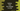# Find average of numbers in a list using python## Calculate the average of numbers in a list using python:

In this tutorial, we are going to show you three different ways to calculate the average of numbers of a list in python. We are using python3 (version 3.6.1). (You can check your python3 version by running python3 –version command on a terminal window). Following are the steps we are going to use in the program :

1. Our program will get all the inputs from the user.
2. The program will ask the user to enter the input numbers separated by comma (’,‘). For example, if the user wants to find out the average of 1,2 and 3, then he will have to enter ’1,2,3’ on the terminal.
3. It will create one list with these input numbers.
4. To create the list, first, the program will split the comma separated numbers using the split() method. It returns one list with all the numbers in it.
5. To find the average of all the numbers in the list, divide the sum of all numbers by the length of the list. We can get the sum of all elements and length of a list by using sum(listname) and len(listname) methods respectively, where listname is the given list.

## Three different ways to calculate the average of list elements in python :

``````#Normal Method

numberList = []
print("Enter all numbers with ',' as separator")

numberList = [int(i) for i in input().split(',')]
print("Average = ", sum(numberList)/len(numberList))

#Statistics module

from statistics import mean

numberList = []

print("Enter all numbers with ',' as separator")
numberList = [int(i) for i in input().split(',')]
print("Average = ", mean(numberList))

#using reduce

from functools import reduce

numberList = []

print("Enter all numbers with ',' as separator")
numberList = [int(i) for i in input().split(',')]
print("Average = ", reduce(lambda x, y: x+y, numberList)/len(numberList))``````

You can also download these programs from here.

### 1. Normal method :

In this example, numberList is the list created by taking the user provided numbers. We have used only one line to read the user input, split these inputs, and convert them to integer while creating the list. That’s the beauty of python 😃

The final average value is calculated by dividing the total sum by the total number of elements in the list. The ‘sum’ method is used to find out the sum of all numbers in the list and len() method is used to find out the length of the list.

### 2. Using ‘statistics’ module :

statistics module contains mathematical statistics functions like mean, median, harmonic mean etc. We can use this module to find out the average or mean of all numbers. We don’t have to import the full module to find out the average of a list as shown in the below program :

As you can see that we are using the ‘mean’ method of ‘statistics’ module to find out the average of a list. We are importing only the ‘mean’ from the ’statistics’ module in the beginning.

### 3. Using reduce :

reduce is a very useful function to work with list elements without iterating it with a loop. We can pass a lambda or rule to it and it will return the final result by doing a rolling computation on the list value.

Here, we will pass one lambda x,y: x+y to reduce with the element list as an argument. It will calculate the sum of all elements of the list using the above lambda. ‘reduce’ is in ‘functools’ module. The length of the list is calculated similarly using the ’len’ method.

### Conclusion :

In this tutorial, we have learned three different ways to find out the average value of a list of numbers. Try to run the programs shown above.

If you know any other ways to calculate the average or if you have any doubt, please leave a comment below.